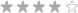#### Currency - All prices are in AUD

##### Currency - All prices are in AUDLoading... Please wait...

# Consumables

• R870.00 (inc VAT) R756.52 (ex VAT)•• R185.00 (inc VAT) R160.87 (ex VAT)• R300.00 (inc VAT) R260.87 (ex VAT)• R440.00 (inc VAT) R382.61 (ex VAT)• R400.00 (inc VAT) R347.83 (ex VAT)• R225.00 (inc VAT) R195.65 (ex VAT)• R84.00 (inc VAT) R73.04 (ex VAT)• R45.95 (inc VAT) R39.96 (ex VAT)• R70.88 (inc VAT) R61.63 (ex VAT)• R216.25 (inc VAT) R188.04 (ex VAT)• R220.00 (inc VAT) R191.30 (ex VAT)• R70.88 (inc VAT) R61.63 (ex VAT)• R1,379.00 (inc VAT) R1,199.13 (ex VAT)•• R220.00 (inc VAT) R191.30 (ex VAT)• R678.00 (inc VAT) R589.57 (ex VAT)• R370.00 (inc VAT) R321.74 (ex VAT)• R172.00 (inc VAT) R149.57 (ex VAT)• R330.00 (inc VAT) R286.96 (ex VAT)• R82.00 (inc VAT) R71.30 (ex VAT)• R2,064.00 (inc VAT) R1,794.78 (ex VAT)• R185.00 (inc VAT) R160.87 (ex VAT)